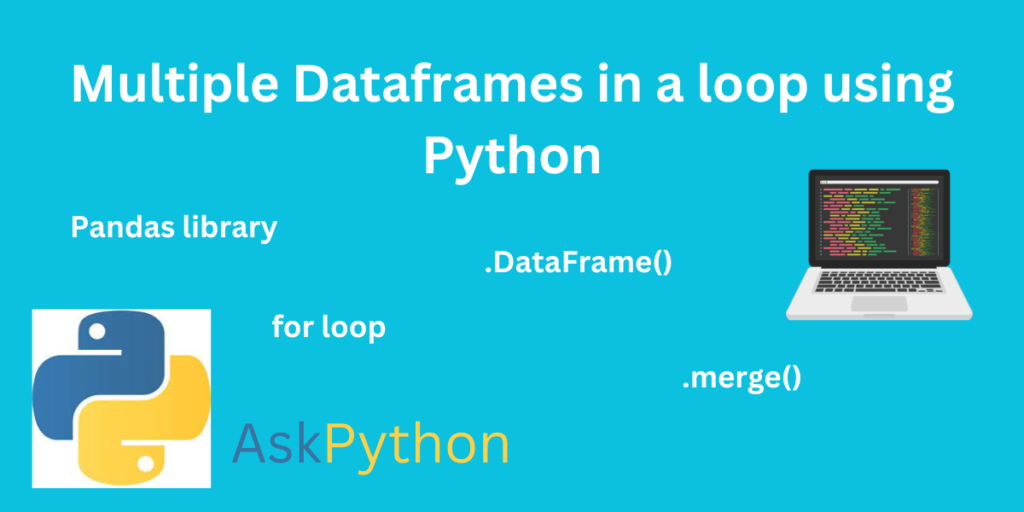# Multiple Dataframes in a Loop Using PythonPandas library is used to create dataframes in python. Let’s understand the process of creating multiple dataframes in a loop using Python. Data frames are used like containers in python to store the data.

These dataframes are used in different applications which are related to different domains like Machine learning, Data science, and Data analytics. If we have a large amount of data in the scattered format, it’ll be very inconvenient to operate. For this purpose, Python provides an excel structure in dataframes. So, it becomes easy to visualize and analyze the data in the form of tables.

## What Is a Dataframe?

A dataframe is a simple container to hold the data in the form of tables. The pandas’ library creates this data frame in a single line of code. Dataframes provide excel like structure for visualization. The data frames are considered as a Two-dimensional and changeable form of container to hold the data. We can add any type of data in the single data frame like string, int, float, or any other type of data at the same time.

This dataframe provides a similar structure like excel to represent the data. For example, the data are always in the columns and rows format. If we want to create a simple table-like structure in excel, we can do that manually. Let’s see the example.

It is very simple in excel to create this table and represent the data. Python also provides a similar structure to represent this data in tabular form.

### How to Create a Dataframe?

Pandas’ library is used to create the dataframe in python. The ‘.DataFrame()’ helps to form dataframes in the pandas’ library. Let’s see the below example for a detailed implementation of dataframes.

```import pandas as pd
Test_Data = [1,2,3,4,5,6,7]
Data_Frame = pd.DataFrame( Test_Data, columns=['Index'])
print(Data_Frame)
```

Here, Test_Data is the list of elements and Data_Frame is a variable that contains the dataframe.

Now we can analyze the similarity between excel and dataframe functions. Both are working in a similar manner.

## Multiple Dataframes in a Python

If we have a large amount of data that need to visualize in the tabular format, then we can use the ‘.merge()’ function. The exact meaning and working of the ‘.merge()’ function is to combine different dataframes and form a new dataframe.

## Application of Multiple Dataframes

Multiple dataframes are used to handle large amounts of data in the Machine Learning and Data Science domain. Take a simple example of an analysis of students in class. If we want to collect information related to students in a tabular format, in this case, the visualization becomes easy with multiple dataframes. The information of students will be inserted in the form of columns. Consider, the columns of the city, phone number, name, grades, parents’ name, and address. So, the information will be displayed as rows and columns structure.

## merge() function for multiple dataframes

Merge() function is used to combine different dataframes. For example, if we have two dataframes we can print that dataframes as a part of the same table. Let’s see the example.

```import pandas as pd
D1 = pd.DataFrame({
'id':[1,2,3,4,5],
'Name': ['A', 'B', 'C', 'D', 'E'],
'subject_id':['Math','English','History','Science','Geography']})
D2 = pd.DataFrame({
'id':[1,2,3,4,5],
'Name': ['F', 'G', 'H', 'I', 'J'],
'subject_id':['Math','English','History','Science','Geography']})
print (pd.merge(D1,D2,on='id'))
```

The first pandas’ library is imported. First, create D1 and D2 (two dataframes) with 2 columns with the same IDs. These dataframes are created using the ‘.DataFrame()’ function. For representing these dataframes as a single/combined dataframe ‘.merge()’ function is used in the code.

In this example, Two different dataframes are combined to form a new dataframe. In this way, we can combine multiple dataframes and represent them as a single dataframe. This is one way to combine multiple dataframes using the merge() function. The only difference between the ‘.merge()’ and ‘.DataFrame()’ functions is ‘.merge()’ is used to combine the dataframes and on the other hand, ‘.DataFrame()’ is for the creation of dataframe in Python.

## Creating Multiple Dataframes Using Loop in Python

Now let’s, see the algorithm to create multiple dataframes using for loop. for loop is used to repeat a single statement of the function multiple times. To understand this work let’s, create multiple dataframes for different subjects. For this, we will initialize the list of subjects and an empty dictionary. Dictionary in python helps to hold the data as a key-value pair. After creating a dictionary, the for loop will be applied to the list of subjects to create a unique dataframe. Finally, print the dictionary. Let’s, implement the code!

```import pandas as pd

List = ['Maths','Science','Geography','History','English']

Dictionary = {}

for i in List:
Dictionary[i] = pd.DataFrame()

print("Created multiple dataframes in a loop:\n", Dictionary)
```

In this example, the pandas’ library is first imported then the list and empty dictionary is initialized. The ‘for loop’ is used to create the individual dataframe for the initialized list. So, after printing the dictionary, we can see that empty dataframes are created for each element of the list.

Here, we have not entered any data for each column so it’ll be printed as empty data columns.